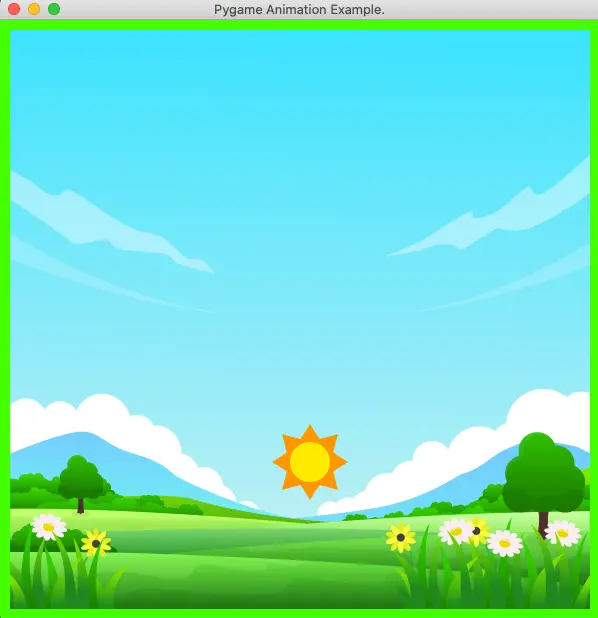# Pygame Animation Example

This example will tell you how to implement animation in pygame game application. It will also tell you how to set the game app’s background image, and how to control the animation speed.

### 1. Pygame Animation Example.

1. Below is the pygame app screenshot. When the app is running, the sun will move along the background image border in the anti-clockwise direction.2. You can see the animation demo video at the end of this article.

### 2. How To Set The Pygame App Main Window Background Image.

1. First, load a background image in pygame.
```BACKGROUND_IMAGE_FILE_PATH = '../../../../img/background-image-blue-sky-2.png'

2. Then draw the above background image surface object to the main window.
```main_window_size = (600, 600)

MAIN_WINDOW_SURFACE = pygame.display.set_mode(main_window_size, pygame.RESIZABLE)

MAIN_WINDOW_SURFACE.blit(BACKGROUND_IMAGE_SURFACE, (10,10))```

### 3. How To Control The Animation Speed.

1. First, we can create an instance of the pygame.time.Clock class.
`FRAME_PER_SECOND_CLOCK = pygame.time.Clock()`
2. Set the frame-per-second value to control the animation speed using the pygame.time.Clock object’s tick() method.
`FRAME_PER_SECOND_CLOCK.tick(60)`
3. The above code will set the frame-per-second value to 60, which means the time between 2 frame paintings on the screen will not be less than 1/60 seconds ( It will not allow drawing more than 60 frames in one second ).

### 4. Pygame Animation Example Source Code.

1. The python source file name is PygameAnimation.py.
2. Below is the PygameAnimation.py source code.
```'''
Created on Feb 6, 2022

@author: songzhao
'''

import pygame, sys

# define the direction constants.
LEFT = 'left'
RIGHT = 'right'
UP = 'up'
DOWN = 'down'

# define the maximum frames per second.
FPS = 60

# define 4 coordinates to represent the image move area border.
MOVE_X_LEFT = 10
MOVE_X_RIGHT = 500
MOVE_Y_TOP = 10
MOVE_Y_BOTTOM = 390

# define the move step value.
MOVE_STEP = 6

# define the pygame app main window surface object variable.
MAIN_WINDOW_SURFACE = None

# this variable is used to save a pygame.time.Clock object.
FRAME_PER_SECOND_CLOCK = None

# the background image surface object.
BACKGROUND_IMAGE_SURFACE = None
BACKGROUND_IMAGE_FILE_PATH = '../../../../img/background-image-blue-sky-2.png'

def initialize_pygame():

pygame.init()

# create the game main window.
main_window_size = (600, 600)

global MAIN_WINDOW_SURFACE
MAIN_WINDOW_SURFACE = pygame.display.set_mode(main_window_size, pygame.RESIZABLE)

# set the window title.
window_title = 'Pygame Animation Example.'
pygame.display.set_caption(window_title)

# create a pygame.time.Clock object.
global FRAME_PER_SECOND_CLOCK
FRAME_PER_SECOND_CLOCK = pygame.time.Clock()

# load the application main window's background image.
global BACKGROUND_IMAGE_SURFACE

# set the frame count in one seconds.
FRAME_PER_SECOND_CLOCK.tick(FPS)

def move_image():

# define the image file path.
image_file_path = '../../../../img/icons8-summer-96.png'
# load the image of the sun.
# define the image's initial coordinates.
img_x = 10
img_y = 10
# define the initial moving direction.
direction = LEFT

# define a variable to record the last time when print the frame on the screen.
last_time = None

# the main loop of the game
while True:

# move to right.
if direction == RIGHT:
img_x += MOVE_STEP
# if reach the main window right border then move up.
if img_x >= MOVE_X_RIGHT:
direction = UP

# move down.
elif direction == DOWN:
img_y += MOVE_STEP
# if reach the main window bottom border then move to right.
if img_y >= MOVE_Y_BOTTOM:
direction = RIGHT

# move to left.
elif direction == LEFT:
img_x -= MOVE_STEP
# if reach the window left border then move down.
if img_x <= MOVE_X_LEFT:
direction = DOWN

# move up.
elif direction == UP:
img_y -= MOVE_STEP
# if reach the window top border then move to left.
if img_y <= MOVE_Y_TOP:
direction = LEFT

# paint the main window background color to green.
MAIN_WINDOW_SURFACE.fill(pygame.Color('green'))

# draw the main window background image.
MAIN_WINDOW_SURFACE.blit(BACKGROUND_IMAGE_SURFACE, (10,10))

# draw the sun image to a new location.
MAIN_WINDOW_SURFACE.blit(img, (img_x, img_y))

# loop in all events and capture the exit event.
for event in pygame.event.get():
# if the catched event is quit.
if event.type == pygame.QUIT:
# then quit the pygame app.
pygame.quit()
sys.exit()

# update the screen to reflect the above draw actions.
pygame.display.update()

# get the current time in milliseconds.
current_time = pygame.time.get_ticks()

if last_time != None:
# get the delta time between 2 frame drawing.
delta_time = current_time - last_time

print('delta_time = ', delta_time)

# assign the current_time variable value to the last_time variable.
last_time = current_time

if __name__ == '__main__':

initialize_pygame()

move_image()```

### 5. Pygame Animation Example Demo Video.

This site uses Akismet to reduce spam. Learn how your comment data is processed.